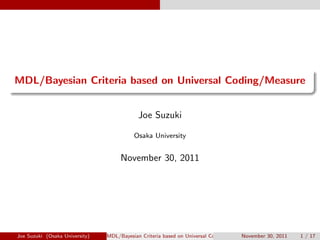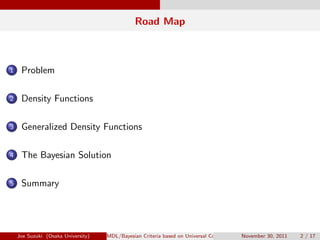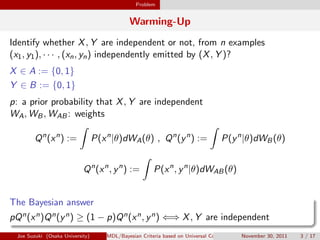Successfully reported this slideshow.

# MDL/Bayesian Criteria based on Universal Coding/Measure

0

Share×
1 of 17
1 of 17

# MDL/Bayesian Criteria based on Universal Coding/Measure

0

Share

MDL/Bayesian Criteria based on Universal Coding/Measure
Joe Suzuki
Solomonoff 85 conference, November 2011

MDL/Bayesian Criteria based on Universal Coding/Measure
Joe Suzuki
Solomonoff 85 conference, November 2011

## More Related Content

### Related Books

Free with a 14 day trial from Scribd

See all

### Related Audiobooks

Free with a 14 day trial from Scribd

See all

### MDL/Bayesian Criteria based on Universal Coding/Measure

1. 1. . ...... MDL/Bayesian Criteria based on Universal Coding/Measure Joe Suzuki Osaka University November 30, 2011 Joe Suzuki (Osaka University) MDL/Bayesian Criteria based on Universal Coding/MeasureNovember 30, 2011 1 / 17
2. 2. Road Map ...1 Problem ...2 Density Functions ...3 Generalized Density Functions ...4 The Bayesian Solution ...5 Summary Joe Suzuki (Osaka University) MDL/Bayesian Criteria based on Universal Coding/MeasureNovember 30, 2011 2 / 17
3. 3. Problem Warming-Up Identify whether X, Y are independent or not, from n examples (x1, y1), · · · , (xn, yn) independently emitted by (X, Y )? X ∈ A := {0, 1} Y ∈ B := {0, 1} p: a prior probability that X, Y are independent WA, WB, WAB: weights Qn (xn ) := ∫ P(xn |θ)dWA(θ) , Qn (yn ) := ∫ P(yn |θ)dWB(θ) Qn (xn , yn ) := ∫ P(xn , yn |θ)dWAB(θ) . The Bayesian answer .. ......pQn(xn)Qn(yn) ≥ (1 − p)Qn(xn, yn) ⇐⇒ X, Y are independent Joe Suzuki (Osaka University) MDL/Bayesian Criteria based on Universal Coding/MeasureNovember 30, 2011 3 / 17
4. 4. Problem Today’s Exercise Identify whether X, Y are independent or not, from n examples (x1, y1), · · · , (xn, yn) independently emitted by (X, Y )? X ∈ A := [0, 1) Continuous Y ∈ B := {1, 2, · · · } Discrete and Inﬁnite . Problem .. ......Construct something like Qn(xn), Qn(yn), Qn(xn, yn). Extend those quantities for general X, Y without assuming either discrete or continuous Joe Suzuki (Osaka University) MDL/Bayesian Criteria based on Universal Coding/MeasureNovember 30, 2011 4 / 17
5. 5. Problem Why Qn (xn ), Qn (yn ), Qn (xn , yn ) can be probabilities? W ∗ A, W ∗ B, W ∗ A,B: the true priors Pn (xn ) := ∫ P(xn |θ)dW ∗ A(θ) , Pn (yn ) := ∫ P(yn |θ)dW ∗ B(θ) Pn (xn , yn ) := ∫ P(xn , yn |θ)dW ∗ AB(θ) Known Use W ∗ A, W ∗ B, W ∗ A,B to compare pPn(xn)Pn(yn) and (1 − p)Pn(xn, yn) Unknown Use WA, WB, WA,B to compare pQn(xn)Qn(yn) and (1 − p)Qn(xn, yn) . The main Issue .. ......What Qn is qualiﬁed to be an alternative to Pn? Joe Suzuki (Osaka University) MDL/Bayesian Criteria based on Universal Coding/MeasureNovember 30, 2011 5 / 17
6. 6. Problem What is the exact Qn for ﬁnite A? P(X = 1) = θ, P(X = 0) = 1 − θ If we weight w(θ) = 1 Kθa(1 − θ)a , K := ∫ dθ θa(1 − θ)a with a > 0, then for each xn = (x1, · · · , xn) ∈ An Qn (xn ) := ∫ w(θ)P(xn |θ)dθ = Γ(2a) ∏ x∈A Γ(cn[x] + a) Γ(a)2Γ(n + 2a) ci [x]: the # of x ∈ A in xi = (x1, · · · , xi ) ∈ Ai Γ: the Gamma function Joe Suzuki (Osaka University) MDL/Bayesian Criteria based on Universal Coding/MeasureNovember 30, 2011 6 / 17
7. 7. Problem Universal Coding/Measures If we choose a = 1/2 (Krichevsky-Troﬁmov) and xn is i.i.d. emitted by Pn (xn ) = n∏ i=1 P(xi ) then, for any P, almost surely, − 1 n log Qn (xn ) → H := ∑ x∈A −P(x) log P(x) From the law of large numbers (Shannon McMillian Breiman): for any P, almost surely, − 1 n log Pn (xn ) = 1 n n∑ i=1 − log P(xi ) → E[− log P(xi )] = H Joe Suzuki (Osaka University) MDL/Bayesian Criteria based on Universal Coding/MeasureNovember 30, 2011 7 / 17
8. 8. Problem The Essential Problem For any P, almost surely, 1 n log Pn(xn) Qn(xn) → 0 (1) (the basis why Pn can be replaced by Qn) . X is neither discrete nor continuous .. ......Into what can Qn and (1) be generalized ? Joe Suzuki (Osaka University) MDL/Bayesian Criteria based on Universal Coding/MeasureNovember 30, 2011 8 / 17
9. 9. Density Functions If X has a density function A: the range of X A0 := {A} Ak+1 is a reﬁnement of Ak Example 1: if A0 = {[0, 1)}, the histogram sequence can be A1 = {[0, 1/2), [1/2, 1)} A2 = {[0, 1/4), [1/4, 1/2), [1/2, 3/4), [3/4, 1)} . . . Ak = {[0, 2−(k−1)), [2−(k−1), 2 · 2−(k−1)), · · · , [(2k−1 − 1)2−(k−1), 1)} . . . sk : A → Ak, sn k : An → An k λ: Lebesgue measure, λn (sn k (xn )) = n∏ i=1 λ(sk(xi )) Joe Suzuki (Osaka University) MDL/Bayesian Criteria based on Universal Coding/MeasureNovember 30, 2011 9 / 17
10. 10. Density Functions {ωk}∞ k=1: ∑ ωk = 1, ωk > 0 gn k (xn ) := Qn k (sn k (xn)) λn(sn k (xn)) , gn (xn ) := ∞∑ k=1 ωkgn k (xn ) fk(xn ) := Pn k (sn k (xn)) λn(sn k (xn)) = n∏ i=1 Pk(sk(xi )) λ(sk(xi )) If we choose {Ak} such that fk → f , for any f n, almost surely 1 n log f n(xn) gn(xn) → 0 (2) B. Ryabko. IEEE Trans. on Inform. Theory, 55, 9, 2009. Joe Suzuki (Osaka University) MDL/Bayesian Criteria based on Universal Coding/MeasureNovember 30, 2011 10 / 17
11. 11. Generalized Density Functions Exactly when does density function exist? B: the Borel set ﬁeld of R µ(D): the probabbility of Borel set D . When a density function exists .. ...... The following are equivalent: for each D ∈ B, λ(D) = 0 =⇒ µ(D) = 0 (µ ≪ λ) There exists dµ dλ := f s.t. µ(D) = ∫ t∈D f (t)dλ(t) Joe Suzuki (Osaka University) MDL/Bayesian Criteria based on Universal Coding/MeasureNovember 30, 2011 11 / 17
12. 12. Generalized Density Functions Density Functions in a General Sense . Radon-Nikodum’s Theorem .. ...... The following are equivalent: for each D ∈ B, η(D) = 0 =⇒ µ(D) = 0 (µ ≪ η) There exists dµ dη := f s.t. µ(D) = ∫ t∈D f (t)dη(t) Example 2: µ({j}) > 0, η({j}) := 1 j(j + 1) , j ∈ B := {1, 2, · · · } µ ≪ η µ(D) = ∑ j∈D∩B f (j)η({j}) dµ dη (j) = f (j) = µ({j}) η({j}) Joe Suzuki (Osaka University) MDL/Bayesian Criteria based on Universal Coding/MeasureNovember 30, 2011 12 / 17
13. 13. Generalized Density Functions In this work, ... B1 := {{1}, {2, 3, · · · }} B2 := {{1}, {2}, {3, 4, · · · }} . . . Bk := {{1}, {2}, · · · , {k}, {k + 1, k + 2, · · · }} . . . sk : B → Bk, sn k : Bn → Bn k gn k (yn ) := Qn k (sn k (yn)) ηn(sn k (yn)) , gn (yn ) := ∞∑ k=1 ωkgn k (yn ) If we choose {Bk} s.t. fk → f , for any f n, almost surely 1 n log f n(yn) gn(yn) → 0 (3) (gn(yn) ∏n i=1 ηn({yi }) is estimation of P(yn) = f n(yn) ∏n i=1 ηn({yi })) Joe Suzuki (Osaka University) MDL/Bayesian Criteria based on Universal Coding/MeasureNovember 30, 2011 13 / 17
14. 14. Generalized Density Functions Joint Density Functions Example 3: A × B (based on Examples 1,2) µ ≪ λη A0 × B0 = {A} × {B} = {[0, 1)} × {{1, 2, · · · }} A1 × B1 A2 × B2 . . . Ak × Bk . . . sk : A × B → Ak × Bk   If {Ak × Bk} satisﬁes fk → f , for any f n, almost surely, we can construct gn s.t. 1 n log f n(xn, yn) gn(xn, yn) → 0 (4) Joe Suzuki (Osaka University) MDL/Bayesian Criteria based on Universal Coding/MeasureNovember 30, 2011 14 / 17
15. 15. The Bayesian Solution If we come back to “Today’s Problem”,... Estimate f n X (xn), f n Y (yn), f n XY (xn, yn) by   gn X (xn), gn Y (yn), gn XY (xn, yn)   . The Bayesian answer .. ......p0gn X (xn)gn Y (yn) ≤ p1gXY (xn, yn) ⇐⇒ X, Y are independent Joe Suzuki (Osaka University) MDL/Bayesian Criteria based on Universal Coding/MeasureNovember 30, 2011 15 / 17
16. 16. The Bayesian Solution In General, ... Givem n example zn and prior {pm} over models m = 1, 2, · · · , estimate f n(zn|m) = dµn dηn (zn |m) w.r.t. model m by gn (zn |m) = dνn dηn (zn |m) s.t. 1 n log dµn dνn (zn |m) → 0 , where µ ≪ η, ν ≪ η, and dµn dνn (zn |m) = dµn dηn (zn |m)/ dνn dηn (zn |m) = f n(zn|m) gn(zn|m) to ﬁnd the model m maxmizing pm · dνn dηn (zn |m) Joe Suzuki (Osaka University) MDL/Bayesian Criteria based on Universal Coding/MeasureNovember 30, 2011 16 / 17
17. 17. Summary Summary and Discussion . Bayesian Measure .. ...... Generalization without assuming Discrete or Continuous Universality as Bayes as well as MDL . Many Applications .. ...... Markov order estimation even when {Xi } is continuous Bayesian network structure estimation Joe Suzuki (Osaka University) MDL/Bayesian Criteria based on Universal Coding/MeasureNovember 30, 2011 17 / 17